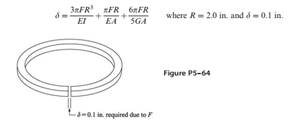### Create an Account

Home / Questions / A piston ring with a split as shown in Figure P5–64 is to be expanded by a tool to facilit...

# A piston ring with a split as shown in Figure P5–64 is to be expanded by a tool to facilitate its installation The ring is sufficiently thin 0.2 in. depth to justify using conventional straight

A piston ring (with a split as shown in Figure P5–64) is to be expanded by a tool to facilitate its installation. The ring is sufficiently thin (0.2 in. depth) to justify using conventional straight-beam bending formulas. The ring requires a displacement of 0.1 in. at its separation for installation. Determine the force required to produce this separation. In addition, determine the largest stress in the ring. Let E = 18 × 106 psi, G = 7 × 106 psi, cross-sectional area A = 0:06 in.2, and principal moment of inertia I = 4:5 × 104 in.4. The inner radius is 1.85 in., and the outer radius is 2.15 in. Use models with 4, 6, 8, 10, and 20 elements in a symmetric model until convergence to the same results occurs. Plot the displacement versus the number of elements for a constant force F predicted by the conventional beam theory equation of Reference .Aug 01 2020 View more View LessSubscribe To Get Solution